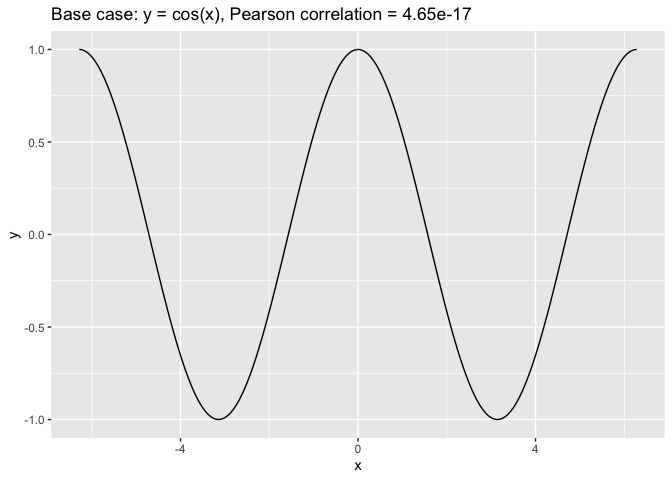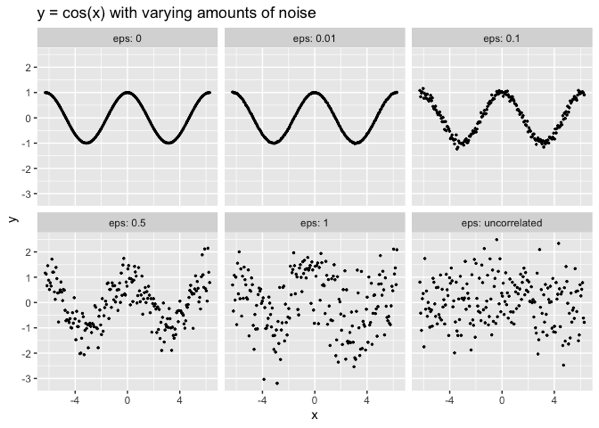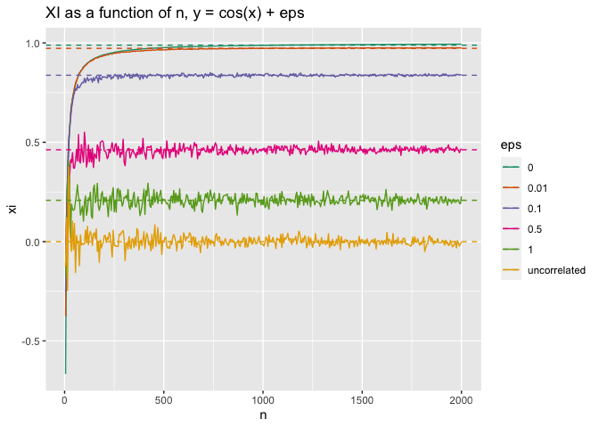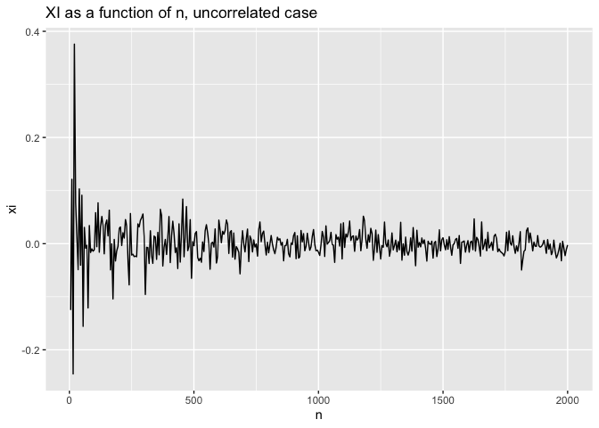# Exploring the XI Correlation Coefficient

Recently, we’ve been reading about a new correlation coefficient, $$\xi$$ (“xi”), which was introduced by Professor Sourav Chatterjee in his paper, “A New Coefficient of Correlation”.

The $$\xi$$ coefficient has the following properties:

• If $$y$$ is a function of $$x$$, then $$\xi$$ goes to 1 asymptotically as $$n$$ (the number of data points, or the length of the vectors $$x$$ and $$y$$) goes to Infinity.
• If $$y$$ and $$x$$ are independent, then $$\xi$$ goes to 0 asymptotically as $$n$$ goes to Infinity.

Unlike traditional correlation coefficients, $$\xi$$ does not assume that the relationship between $$x$$ and $$y$$ is linear (or “linear-ish”); in principle, it can be any functional relationship. This sounds useful! Note, however, that $$\xi$$ is not symmetric, and that $$\xi$$ has no concept of “anti-correlation.” The value of $$\xi$$ can be negative, but this negativity does not have any innate significance (other than being “close to zero,” as we will discuss later).

So perhaps, rather than thinking of $$\xi$$ as a “correlation,” we might find it more useful to think of it as a measure of dependence: specifically, the degree to which $$y$$ is dependent on $$x$$.

In this post, we’ll run through some informal experiments to try to get a sense of what different values of $$\xi$$ might mean.

The questions we are interested in are:

• How large does $$n$$ have to be before we observe the asymptotic estimate?
• What happens when the relationship between $$x$$ and $$y$$ is noisy?
• Can we put a meaningful interpretation on values of $$\xi$$ that are not 0 or 1?

We’ll do our experiments in R, using the calculateXI() function from the XICOR package.

## The Base Case: Functional relationship between $$x$$ and $$y$$

We’ll take as our baseline the case $$y = \cos(x)$$, over two periods. We chose this function because it’s a simple case that linear correlation coefficients can’t handle: there is a clear relationship between $$y$$ and $$x$$, but it is highly non-monotonic (let alone linear). In fact, for our selected range, the linear correlation between $$x$$ and $$\cos(x)$$ is zero.## The Test Case: Functional but noisy relationship between $$x$$ and $$y$$

Now let’s add some noise to the base case; the noise is normally distributed with zero mean and standard deviation $$\epsilon$$. We’ll look at $$\epsilon$$ in the range from 0.01 (very low amplitude noise) to 1 (high amplitude noise, relative to the range of $$\cos(x)$$). We’ll also add the noiseless case, and the completely uncorrelated case, where $$y$$ is normally distributed noise, with no relationship to $$x$$ whatsoever.In addition to varying $$\epsilon$$, we’ll also vary $$n$$, the number of data points, so that we can examine the relationship between $$\xi$$ and the amount of available data.

We’ll also estimate the asymptotic value of $$\xi$$ (as $$n$$ gets larger) for each situation.

The R code to replicate these experiments is here. We use the function XICOR::calculateXI() to calculate $$\xi$$:

# By default, the function sets a random seed
# for reproducible results. seed=NULL
# reinitializes the seed. It's not really what
# I want, but <shrug>.

xi = calculateXI(df$x, df$y, seed=NULL)


Here are the results:Approximate asymptotic values of XI
eps xi
0 0.989
0.01 0.973
0.1 0.837
0.5 0.460
1 0.208
uncorrelated 0.000

From the noiseless and low noise ($$\epsilon = 0.01$$) cases, we can see that $$\xi$$ asymptotes to a value very near 1 for $$n > 500$$. In fact, I’d say that $$n$$ larger than about 250 is sufficient to get a good estimate of $$\xi$$ in the situations that we are looking at.

As the relationship between $$x$$ and $$y$$ gets noisier, $$\xi$$ gets smaller, but still converges to a value bounded away from zero. The general interpretation seems to be that the closer $$\xi$$ is to 1, the stronger the functional relationship between $$x$$ and $$y$$ — but the important thing is that $$\xi$$ be bounded away from zero (and positive).

In the uncorrelated case, $$\xi$$ converges to a value very near zero.

## The uncorrelated case

Let’s take a closer look at the uncorrelated case as $$n \to \infty$$.The first observation is that $$\xi$$ is negative about half the time in this experiment. Overall, $$\xi$$ varies symmetrically around zero, with the bandwidth of the variation decreasing as $$n$$ increases. This is consistent with Theorem 2.1 of Professor Chatterjee’s paper:

Suppose that $$X$$ and $$Y$$ are independent and $$Y$$ is continuous. Then √$$n$$ ξ → $$N(0, 2/5)$$ in distribution as $$n \to \infty$$

Prof. Chatterjee goes on to say, “It [the convergence] is roughly valid even for $$n$$ as small as 20.” Looking at the chart above, I might still stick to $$n$$ larger than about 250 or so.

## Significance tests

Of course, an actual significance test is more precise than eyeballing a graph. The XICOR::xicor() function provides a significance test for $$\xi$$, under the null hypothesis that $$x$$ and $$y$$ are independent.

Let’s try it on some large and small noisy data sets.

# function to pretty print the results from xicor()
# pval is the threshold for rejecting
# the null hypothesis
xitest = function(x, y, pval) {
xc = xicor(x, y, pvalue=TRUE)
if(xc$pval < pval) decision = 'REJECT' else decision = "DON'T REJECT" print(paste('xi =', xc$xi, ', p =', xc$pval)) print(paste(decision, 'independence of x and y')) } # x and y uncorrelated # tiny dataset, bigger dataset uc_10 = nocorr_set(10) uc_500 = nocorr_set(500) # y noisily dependent on x # tiny dataste, bigger dataset noisy_10 = noisy_simple_set(10, eps=1) noisy_500 = noisy_simple_set(500, eps=1) # threshold for rejecting null hypothesis p = 0.025 ### $$x$$ and $$y$$ uncorrelated Here, we don’t want to reject the hypothesis that $$x$$ and $$y$$ are independent. xitest(uc_10$x, uc_10$y, p) ##  "xi = 0.121212121212121 , p = 0.276677929806404" ##  "DON'T REJECT independence of x and y" xitest(uc_500$x, uc_500$y, p) ##  "xi = -0.00342001368005462 , p = 0.548120682841176" ##  "DON'T REJECT independence of x and y" ### $$y$$ noisily dependent on $$x$$ Here, we’d like to reject the hypothesis that $$x$$ and $$y$$ are independent. xitest(noisy_10$x, noisy_10$y, p) ##  "xi = 0.0606060606060607 , p = 0.383473964035915" ##  "DON'T REJECT independence of x and y" xitest(noisy_500$x, noisy_500\$y, p)
##  "xi = 0.269509078036312 , p = 0"
##  "REJECT independence of x and y"

Notice that the xicor significance test didn’t successfully identify the dependency between $$x$$ and $$y$$ in the tiny ($$n$$ = 10) data set, suggesting $$n$$ less than 10 may be too small to guarantee convergence to $$\xi$$’s asymptotic value with any statistical certainty.

## So what does this all mean?

Informally speaking:

• If $$n$$ is large enough (let’s say greater than 250), and $$\xi$$ is large enough (say, greater than 0.25), then one can probably safely assume that $$y$$ is dependent on $$x$$. Note that I am picking the values for “large enough” by unscientifically eyeballing the graphs above.
• The closer to 1 that $$\xi$$ is (for large enough $$n$$), the stronger the dependency.
• If $$n$$ or $$\xi$$ are fairly small, one should use the $$\xi$$-based test of independence to decide.

Overall, the $$\xi$$ correlation coefficient seems to be an effective and fairly general score for determining the directional dependency of two variables. While the paper does admit that the $$\xi$$-based significance test for independence is not as powerful (in the statistical sense) as other standard methods when the relationship between two variables is smooth and non oscillatory, the method does excel at identifying oscillatory and highly non-monotonic dependencies.

To quote the paper, the relative lack of power in identifying non-oscillatory dependencies “is a matter of concern only when the sample size is small,” which is a situation that tends not to arise in data science and other “large data” applications. Furthermore, the $$\xi$$ calculations are fairly efficient ($$n \log n$$, compared to some more powerful methods, which are $$n^2$$), giving them an advantage in larger-data situations.

There are other aspects of $$\xi$$ that might be considered disadvantages. The calculation is not symmetric; it measures dependency in one direction only. It also doesn’t have a notion of “anti-correlation”: it will tell you if $$y$$ is dependent on $$x$$, but not whether the correlation between them is direct or inverse.

From a data science perspective, the $$\xi$$ correlation and associated significance test may prove to be useful for tasks like variable selection, an application we hope to cover in a future post.### Nina Zumel

Data scientist with Win Vector LLC. I also dance, read ghost stories and folklore, and sometimes blog about it all.

### 2 replies ›

1.Sami says:

Dear Nina,

Your R script is great! Applying this robust index to the cosine curve was inspiring. Many thanks for that. After reading Chatterjee’s paper, I am not sure whether *autocorrelation* is a problem when calculating ξ (“xi”) between two variables in time series. I would appreciate your opinion on this issue.

1.Nina Zumel says:

Thanks for the kind words! As for autocorrelation — since xi works on the rank statistics of the values, rather than time, it seems that autocorrelation wouldn’t be an issue. But I haven’t explored that in depth.